Home > A2C > Chapter 7 > Lesson 7.3.5 > Problem7-226

7-226.
1. Graph each of the following systems of inequalities. Homework Help ✎

1.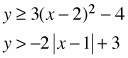2.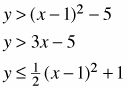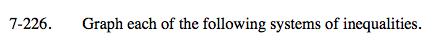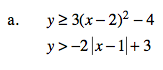First graph the system as if the inequalities were equations.

Test to see if the origin (0,0) works in either of the equations.

Now use this information to fill in the correct part of the graph.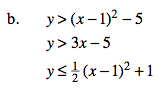See part (a).